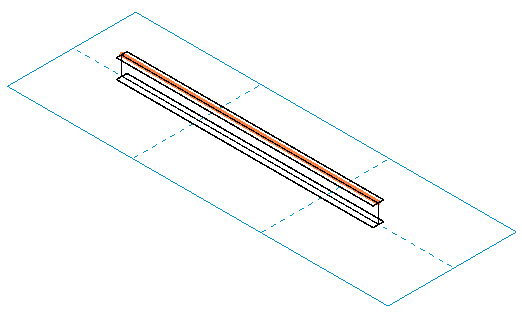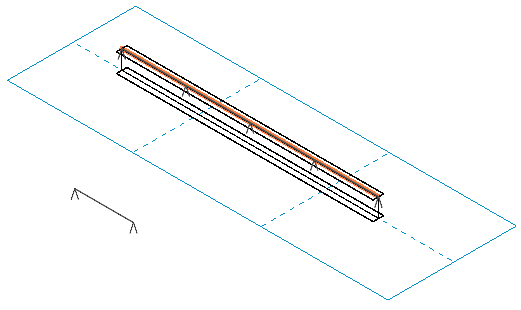In Revit Structure, a new line load is created using the NewLineLoad method. It provides four different overloads, which we wish to explore here in a little more detail:

• NewLineLoad(XYZ, XYZ, XYZ, XYZ, XYZ, XYZ, Boolean, Boolean, Boolean, LineLoadType, SketchPlane): unhosted line load using data at two points.
• NewLineLoad(XYZArray, XYZArray, XYZArray, Boolean, Boolean, Boolean, LineLoadType, SketchPlane): unhosted line load using data at an array of points.
• NewLineLoad(Element, XYZArray, XYZArray, Boolean, Boolean, Boolean, LineLoadType, SketchPlane): hosted line load using data at an array of points.
• NewLineLoad(Reference, XYZArray, XYZArray, Boolean, Boolean, Boolean, LineLoadType, SketchPlane): hosted line load using data at an array of points.

The first few arguments vary, and provide for the different methods of defining the line load location or host element. The last five are always the same:

• Boolean uniform.
• Boolean projected.
• Boolean isReaction.
• SketchPlane.

The arguments differing between the overloads are:

• XYZ p1, XYZ f1, XYZ m1, XYZ p2, XYZ f2, XYZ m2: two points and the forces and moments at each to define an unhosted line load.
• XYZArray points, XYZArray forces, XYZArray moments: arrays to define the points, forces and moments of an unhosted line load.
• Element, XYZArray forces, XYZArray moments: an element and arrays of forces and moments to define a hosted line load.
• Reference, XYZArray forces, XYZArray moments: a curve reference and arrays of forces and moments to define a hosted line load.

The first one takes two triples of XYZ arguments for the point coordinates, force and moment at each of two given points.

The three others take XYZArray arguments to define the forces and moments. The overload taking three arrays, one each for the points, forces and moments, is the only one that can accommodate an arbitrary number greater than one of points, forces and moments.

The last two overloads define hosted line loads. Here, these arrays are expected to have exactly two entries, which can then be distributed evenly from start to end point along the host element or curve. An attempt to add a third entry to the force array, for example, will cause an exception to be thrown.

Jiri Smerak of Ing.-Software Dlubal GmbH has done some further research on how to use these methods. He implemented an external command for testing the various overloads and their parameter combinations. I used his code as a basis to implement a new Building Coder sample command CmdNewLineLoad. It successfully creates:

• An unhosted line load defined by two points.
• A hosted line load on a beam element.
• A hosted line load on a beam's analytical curve.

Jiri tested creating hosted line loads on braces and columns and confirms that that works as well. He also tested the overloads taking a reference parameter, and that works fine on a beam or column's analytical line reference, including arc and nurbs beams.

Here is the code for the external command Execute method:

```Application app = commandData.Application;
Document doc = app.ActiveDocument;

Autodesk.Revit.Creation.Application ca
= app.Create;

Autodesk.Revit.Creation.Document cd
= doc.Create;

Autodesk.Revit.Creation.Filter cf
= ca.Filter;

// determine line load symbol to use:

List<Element> symbols = new List<Element>();
Filter f1 = cf.NewTypeFilter( typeof( LineLoadType ) );
int n = doc.get_Elements( f1, symbols );

= symbols as LineLoadType;

// sketch plane and arrays of forces and moments:

Plane plane = ca.NewPlane( XYZ.BasisZ, XYZ.Zero );
SketchPlane skplane = cd.NewSketchPlane( plane );

XYZ forceA = new XYZ( 0, 0, 5 );
XYZ forceB = new XYZ( 0, 0, 10 );
XYZArray forces = new XYZArray();
forces.Append( forceA );
forces.Append( forceB );

XYZ momentA = new XYZ( 0, 0, 0 );
XYZ momentB = new XYZ( 0, 0, 0 );
XYZArray moments = new XYZArray();
moments.Append( momentA );
moments.Append( momentB );

BuiltInCategory bic
= BuiltInCategory.OST_StructuralFraming;

f1 = cf.NewTypeFilter( typeof( FamilyInstance ) );
Filter f2 = cf.NewCategoryFilter( bic );
Filter f3 = cf.NewLogicAndFilter( f1, f2 );

List<Element> beams = new List<Element>();

n = doc.get_Elements( f3, beams );

XYZ p1 = new XYZ( 0, 0, 0 );
XYZ p2 = new XYZ( 3, 0, 0 );
XYZArray points = new XYZArray();
points.Append( p1 );
points.Append( p2 );

// create a new unhosted line load on points:

points, forces, moments,
false, false, false,

Debug.Print( "Unhosted line load works." );

// create new line loads on beam:

foreach( Element e in beams )
{
try
{
e, forces, moments,
false, false, false,

Debug.Print( "Hosted line load on beam works." );
}
catch( Exception ex )
{
Debug.Print( "Hosted line load on beam fails: "
+ ex.Message );
}

FamilyInstance i = e as FamilyInstance;

AnalyticalModelFrame amFrame
= i.AnalyticalModel as AnalyticalModelFrame;

foreach( Curve curve in amFrame.Curves )
{
try
{
curve.Reference, forces, moments,
false, false, false,

Debug.Print( "Hosted line load on "
+ "AnalyticalModelFrame curve works." );
}
catch( Exception ex )
{
Debug.Print( "Hosted line load on "
+ "AnalyticalModelFrame curve fails: "
+ ex.Message );
}
}
}
return CmdResult.Succeeded;
```

The command creates one free-standing line load on a pair of given points. It also picks up all family instances with the structural framing category and creates line loads on both the elements and their analytical model curves. I ran it this minimal project containing one single beam on a work plane grid:This is the report resulting from running the command in this minimal sample project:

```Unhosted line load works.
Hosted line load on beam works.
Hosted line load on AnalyticalModelFrame curve works.
```

Here you see the line loads added, one on the given points and the other two hosted by the beam:Here is version 1.1.0.41 of the complete Visual Studio solution including the new command.

Thank you very much Jiri for all your research!